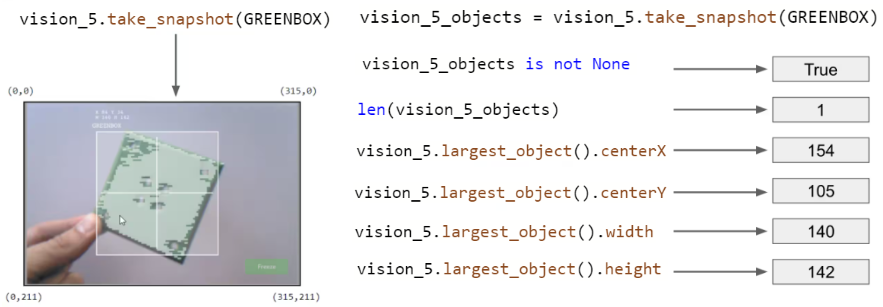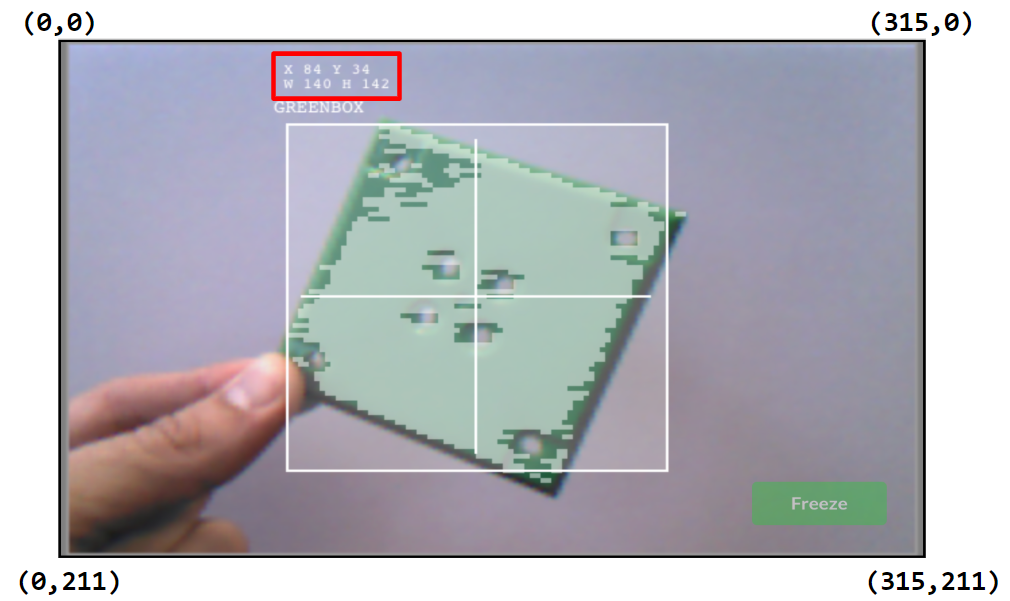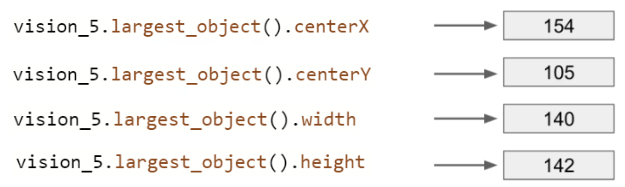STEMLabs V5
Vision Sensor Student

### The Vision Sensor's Sensing Instructions

VEXcode V5 has Sensing instruction for the Vision Sensor. The first two you already used in the Play section to take a snapshot and to check if the object exists.

In the figure below, you see that the snapshot captured the GREENBOX snapshot. The object, GREENBOX, was identified in the snapshot and so the answer of whether it exists is TRUE.

Let's look at these other Sensing instructions and what their values tell us.• The len function and the take_snapshot instruction tell us how many GREENBOX objects are in the snapshot. Here, there is only 1 detected.

• The center X value tells us whether the GREENBOX object is to the left or right of the robot's center point. Remember, the Vision Sensor should be mounted in the middle of the robot facing forward and so the snapshot's view is the robot's view.

• If center X is greater than 157.5, the object is to the right of the robot's center point.

• If center X is less than 157.5, the object is to the left of the robot's center point.

• The center Y value tells us whether the GREENBOX is higher or lower than the robot's center point.

• If center Y is greater than 105.5, the object is lower than the robot's center point.

• If center Y is less than 105.5, the object is higher than the robot's center point.

• The width and height values tell us how close the GREENBOX is to the robot.

• The same-sized object will be larger in width and height as it gets closer to the robot.

### How are the center X and center Y values calculated?

The values are calculated based on the coordinates within the snapshot. The width and height of the object are already calculated.

The Vision Sensor tracks the X and Y values of the upper left corner of the object. Below, those coordinates are (84, 34).The center X and center Y values can be calculated based off of the coordinates of the upper left corner (84, 34), and the width (W 140) and height (H 142) values provided.• centerX = 140/2 + 84 = 154

• centerX = half the width of the object added to its leftmost X coordinate

• centerY = 142/2 + 34 = 105

• centerY = half the height of the object added to its topmost Y coordinate